# Introduction To Graphs NCERT Solutions : Class 8 Maths

## NCERT Solutions for Class 8 Maths : Introduction To GraphsFilter
Filters :
Classes
•
•
•
•
•
•
•
Chapters
•
•
•
•
•
•
•
•
•
•
• 6 More
Exercises
•
•
•
•

### NCERT Class 8 | INTRODUCTION TO GRAPHS | Exercise 01 | Question No. 04

The following graph shows the temperature forecast and the actual temperature for each day of a week. (a)  On which days was the forecast temperature the same as the actual temperature? (b)  What was the maximum forecast temperature during the week? (c)  What was the minimum actual temperature during the week? (d)  On which day did the actual temperature differ the most from the forecast temperature?### NCERT Class 8 | INTRODUCTION TO GRAPHS | Exercise 01 | Question No. 05

Use the tables below to draw linear graphs. (a) The number of days a hill side city received snow in different years. Years 2003, 2004, 2005 and 2006 and Days 8, 10, 5, and 12 (b) Population (in thousands) of men and women in a village in different years.### NCERT Class 8 | INTRODUCTION TO GRAPHS | Exercise 01 | Question No. 06

A courier-person cycles from a town to a neighbouring suburban area to deliver a parcel to a merchant. His distance from the town at different times is shown by the following graph. (a)  What is the scale taken for the time axis? (b)  How much time did the person take for the travel? (c)  How far is the place of the merchant from the town? (d)  Did the person stop on his way? Explain. (e)  During which period did he ride fastest?### NCERT Class 8 | INTRODUCTION TO GRAPHS | Exercise 03 | Question No. 02

Draw a graph for the following. (i) Side of square (in cm) 2, 3,3.5,5,6 and Perimeter (in cm) 8, 12, 14, 20, 24 Is it a linear graph ? (ii) Side of square (in cm) 2, 3, 4, 5, 6 and Area (in cm) 4, 9, 16, 25, 36 Is it a linear graph ?Doubtnut one of the best online education platform provides free NCERT Solutions of Maths for Class 8 Introduction To Graphs which are solved by our maths experts as per the NCERT (CBSE) guidelines. We provide the solutions as video solutions in which concepts are also explained along with answer which will help students while learning , preparing for exams and in doing homework. These Solutions will help to revise complete syllabus and score more marks in examinations.

Get here free, accurate and comprehensive NCERT Solutions for Class 8 Maths Introduction To Graphs which have been reviewed by our maths counsellors as per the latest edition following up the CBSE guidelines. We provide video solutions in which solutions to all the questions of NCERT Class 8 Maths textbook are explained in step by step and detailed way.

## The Topics of the Chapter Introduction To Graphs are :

INTRODUCTION TO GRAPHS

CONSTRUCTION OF GRAPHS

PLOTTING OF POINTS

It contains these exercises along with solved examples. All the exercises are solved in the video. Select the exercise to view the solutions exercisewise of the Chapter Introduction To Graphs:

## NCERT Solutions Class 8 Maths Chapter Introduction To Graphs Exercises:

We have covered all the exercises and also Solved examples in the videos. Along with the practise exercise Students should also practise solved examples to clear the concepts of the Introduction To Graphs. If incase you have any doubt you can watch the solutions for the given questions.Watch the solutions for the given questions in which it is also explained in the video steps to solve the questions along with answers.

NCERT Class 8 Maths Solutions Chapter 15 Introduction to Graphs

In this chapter, you will be made familiar with the graphical representation of data.  Some of the topics that are covered in this chapter include Bar Graph, Pie chart (or a circle-graph), histogram, line graph, linear graphs, etc. Students are required to get a clear idea of how to utilize these graphs in the right manner. Important topics like how to locate a point as well as finding the coordinates have been covered. Doubtnut has provided lots of figures to make the students get the right idea about the different topics in the perfect manner.

NCERT books are useful for the students to get the basic concepts cleared.  At the same time, it fails to provide detailed explanations of the different topics and subtopics. So, students of Maths are not able to score good marks at all for which they lose their confidence. With the aim to get their queries cleared and achieve success in the exams, they try to look out for different reference guides.

All these problems faced by the students have been taken seriously by the expert tutors of Doubtnut. So, they have come up with the best solutions to make the students get the right and clear picture of the different topics. Students who wish to get an in-depth explanation of the subject can look forward to opting for the best online study material provided by experienced teachers.  All efforts have been made to provide the students with a step-by-step procedure that makes it much easier for the students to get the right idea about it. These video tutorials are also free to access by the students where there are no fees for it. Doubtnut has also provided lots of examples that have made it possible for the students to get the right practice done. It has also gone a step ahead by providing Class 8 Maths NCERT Solutions as well.

## Topics and Subtopics included in NCERT Solutions for Class 8 Maths Chapter 15 Introduction to Graphs

Introduction: You have noticed lots of graphs in books, newspapers, magazines, television, etc? Well, showing numerical facts in visual form in order to get the right and the clear idea is the main purpose of the graphs. One can also try to represent data in the form of a table but it is much easier to understand in the form of a graphical representation.  So, let’s recall some types of graphs:

### A Bar Graph

This type of graph is used in order to show the comparison among categories. You can find two or more parallel horizontal or vertical bars or rectangles. For example, let us have a look at the marks obtained in Mathematics by a particular student in three-terminal examinations.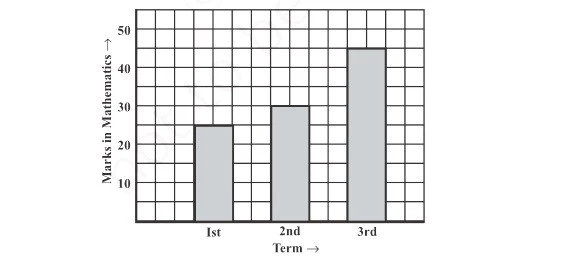### A Pie chart (or a circle-graph)

In order to compare parts of a whole, a pie chart is used. For example, the below pie chart or circle graph shows the different percentage of viewers who watches different types of TV channels.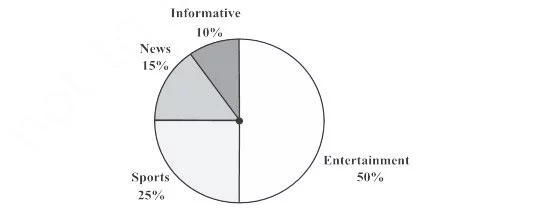### A histogram

A bar graph that shows data in intervals is called a histogram. In the below histogram, the weight distribution of 40 persons of a locality has been illustrated.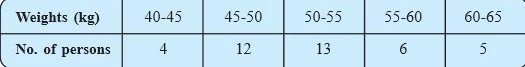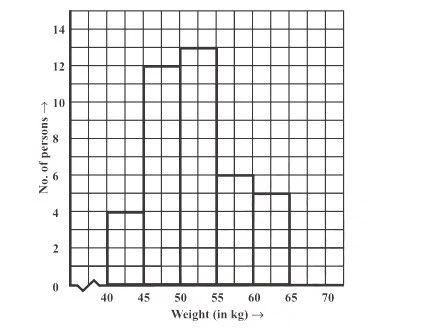### A Line Graph

There are data that keeps changing continuously over periods of time. This type of data is displayed with the help of a line graph. For example, a student fell ill and the doctor advised her family members to check her body temperature every four hours.  Let’s have a look at the time temperature graph as below: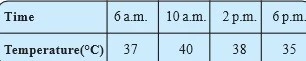The timings are shown in the horizontal line which is known as the x-axis and the temperatures are shown on the vertical line which is called the y-axis. So, you can have a look at the line graph below: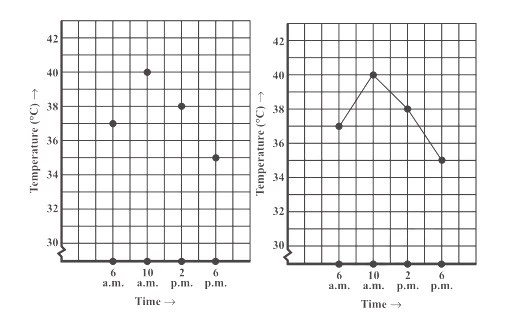### Linear Graphs

When it comes to a line graph, it consists of bits of line segments that are joined consecutively. So, this type of graph is called a linear graph. Here, locating points conveniently is important on a graph sheet.

#### Location of a Point

A dot is put on the blackboard by a teacher. Now the students were questioned to describe its location.  Several locations were given by the students.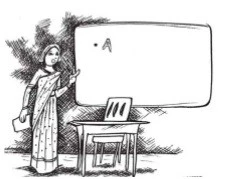One of the students said that the dot is in the upper half of the board. While another student said that the dot is very close to the left upper corner of the board. The third student said that the dot is near the left edge of the board. The right answer was given by the fourth student who said that the dot is160 cm from the bottom edge and 90 cm from the left edge.  So, this was the best and correct answer said by the student.

#### When you book a movie ticket and search for your reserved seat, then you need to have the right information on the row number and the seat number. Let’s have a look at this figure: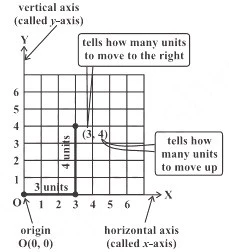Here, you get to have a look at the points 3, 4 that indicates 3 units from the left edge and 4 units from the bottom edge. So, the x-coordinate of the point is 3 while 4 is the y-coordinate of the point.  So, 3, 4 are said to be the coordinates of the point.

### Some Applications

It is a known fact that the more a utility is used, the more we need to pay for it. In summers, more electricity is consumed than winters which lead to an increase in the energy bills. So, one quantity affects another. So, the more you consume, the more you need to pay for it. Thus, the quantity of electricity is said to be an independent or control variable and the amount of the electricity bill is known as the dependent variable.

## Why is Doubtnut useful for Class 8 Maths Students?

Doubtnut has truly revolutionized the way of learning by the students in the best way. With its best video tutorials, students are able to get the right idea on how to work on the different types of graphs.  Let’s have a look at some of the usefulness of Doubtnut for the students:

• Ease of learning: Students do not have to worry whether they are at home or any other place because they can download the course materials online in the form of pdf. This helps them to study in the best way according to their choice. Therefore, Doubtnut’s online study material prove to be a boon for the students where they get the perfect idea on how to work on the different questions.  In other words, they can feel quite confident in this subject.
• Get the best support: The team of Doubtnut helps to provide students with the best support. This helps a lot for the students to get cleared of all their different queries in the right way. Thus, this also leads to achieving good marks in the exams where they do not find it difficult at all to solve any problems. So, this truly proves to be an added advantage for them where they can solve even the toughest question without taking a lot of time at all.
• Lots of exercises and examples included: With lots of exercises and examples provided in the NCERT Class 8 Maths Solutions Chapter 15, you will get all the right idea on how to attempt the different questions in the best and easiest manner.  So, students willing to score good marks in the examinations should definitely make the right choice to get hold of the comprehensive study materials by the expert tutors of Doubtnut.

Share this on :-